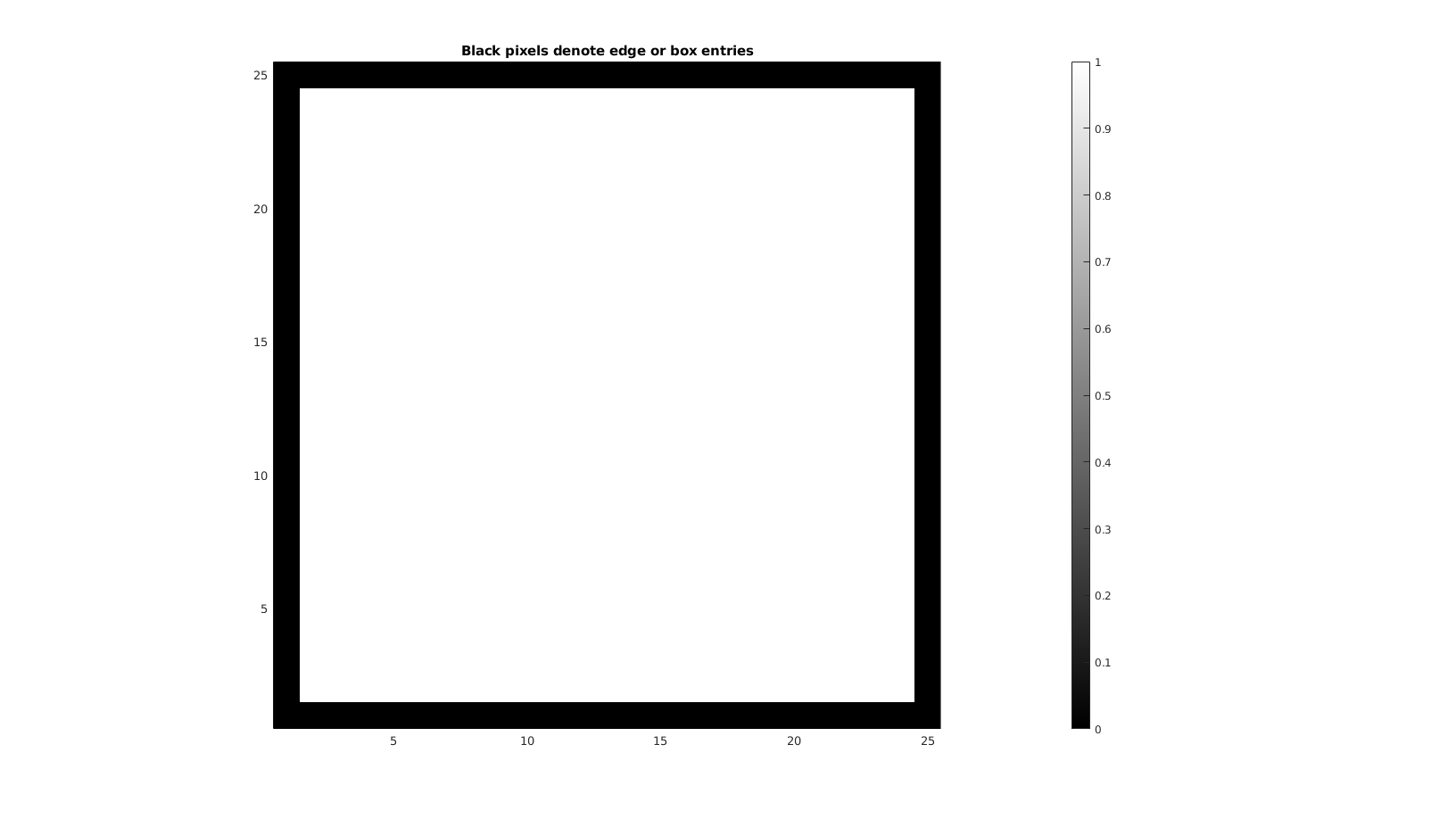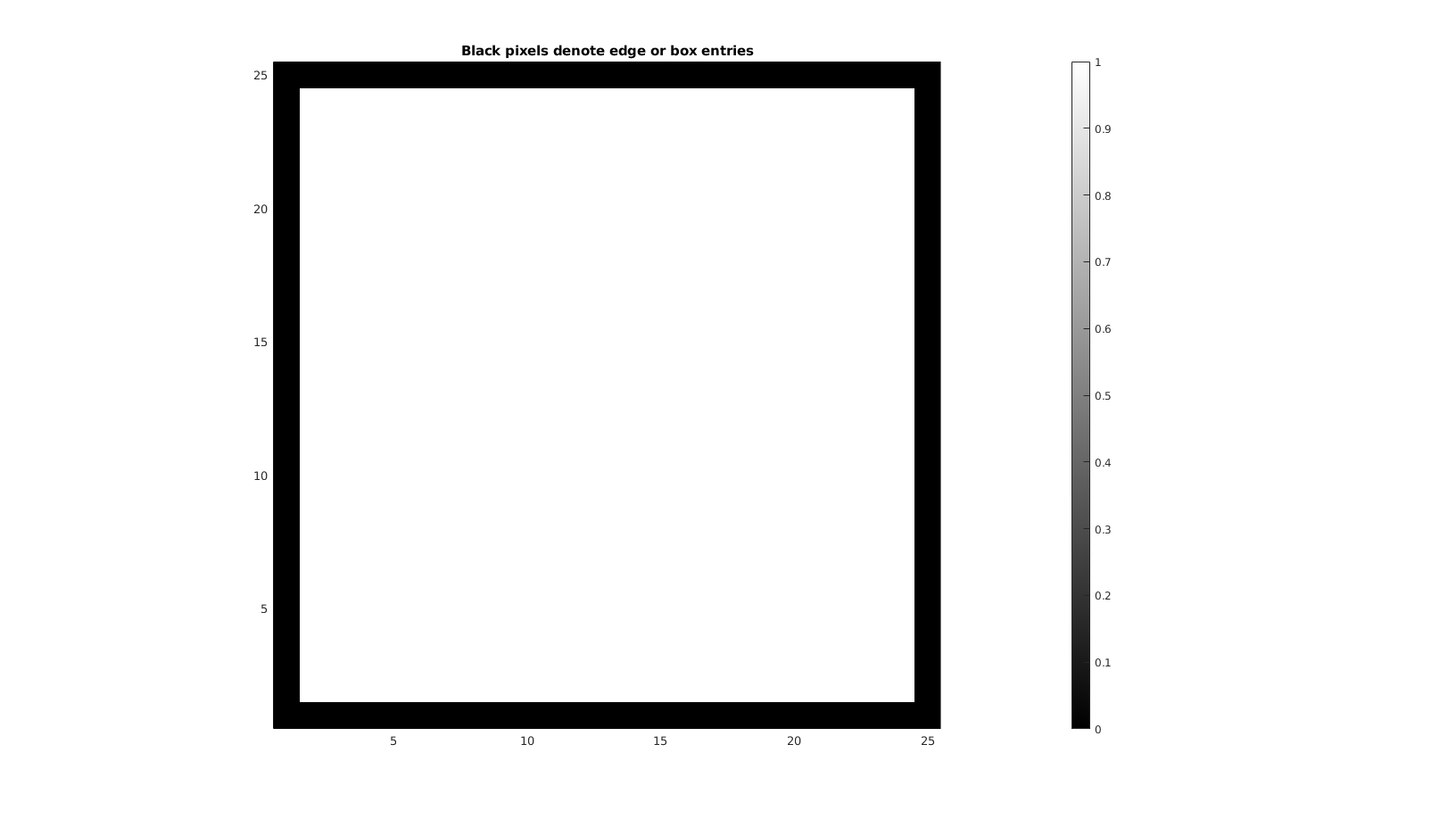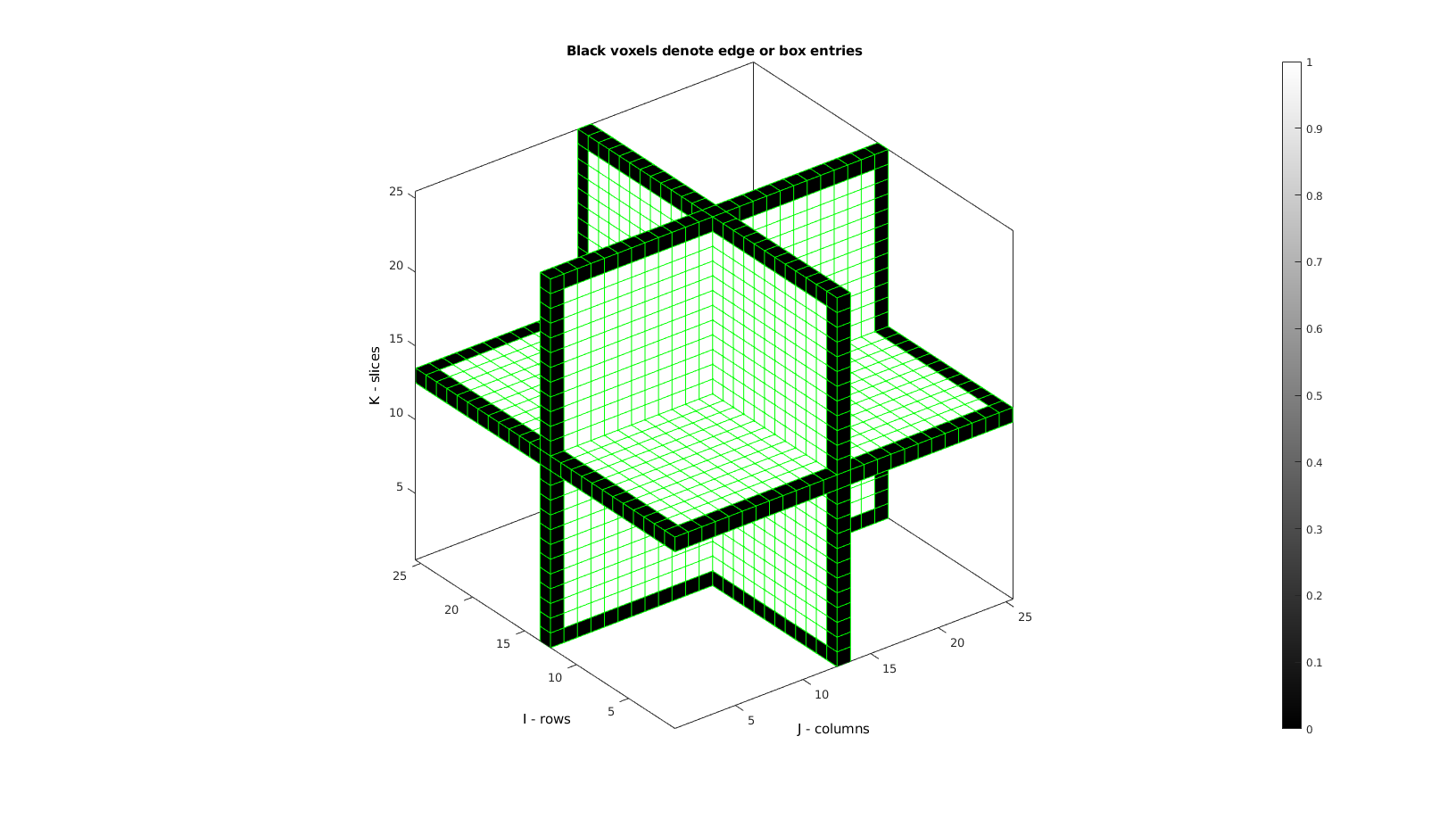# box_indices

Below is a demonstration of the features of the box_indices function

## Syntax

[IND]=box_indices(siz);

## Description

The box_indices function returns the indices of the outer boundary elements of an array (which can be thought of as defining a box), i.e. the indices of the first and last row, columsn, slice, etc..

## Examples

```clear; close all; clc;
```

Plot settings

```faceAlpha1=1;
faceAlpha2=0.65;
edgeColor1='none';
edgeColor2='none';
```

## Example: box_indices for 2D arrays

```siz=[25 25];
M=ones(siz);
[indBox]=box_indices(size(M));
M(indBox)=0; %setting edge indices to 0 for visualization
```

Plotting results

```cFigure;
title('Black pixels denote edge or box entries'); hold on;
imagesc(M);
axis equal; axis tight; axis vis3d; grid off;
colormap gray; caxis([0 1]); colorbar;
drawnow;
```## Example: box_indices for 3D arrays

```siz=[25 25 25];
M=ones(siz);
[indBox]=box_indices(size(M));
M(indBox)=0; %setting edge indices to 0 for visualization
```Plotting results. Visualization shows 3 mutally orthogonal slices but black voxels are actually all around the 3D matrix.

```% Creating patch data for voxel display
logicPlot=false(size(M));
logicPlot(:,:,round(size(M,3)/2))=1;
logicPlot(:,round(size(M,2)/2),:)=1;
logicPlot(round(size(M,1)/2),:,:)=1;
[F,V,C]=ind2patch(logicPlot,M,'v');

% [F2,V2,C2]=ind2patch(M==0,M,'vb');

cFigure;
title('Black voxels denote edge or box entries'); hold on;
xlabel('J - columns');ylabel('I - rows'); zlabel('K - slices'); hold on;

patch('Faces',F,'Vertices',V,'FaceColor','flat','CData',C,'EdgeColor','g','FaceAlpha',1);
% patch('Faces',F2,'Vertices',V2,'FaceColor','flat','CData',C2,'EdgeColor','k','FaceAlpha',0.1);

axis equal; view(3); axis tight; axis vis3d; grid off;  box on;
colormap gray; caxis([0 1]); colorbar;
drawnow;
```GIBBON www.gibboncode.org

Kevin Mattheus Moerman, [email protected]

GIBBON footer text

GIBBON: The Geometry and Image-based Bioengineering add-On. A toolbox for image segmentation, image-based modeling, meshing, and finite element analysis.

Copyright (C) 2019 Kevin Mattheus Moerman

This program is free software: you can redistribute it and/or modify it under the terms of the GNU General Public License as published by the Free Software Foundation, either version 3 of the License, or (at your option) any later version.

This program is distributed in the hope that it will be useful, but WITHOUT ANY WARRANTY; without even the implied warranty of MERCHANTABILITY or FITNESS FOR A PARTICULAR PURPOSE. See the GNU General Public License for more details.

You should have received a copy of the GNU General Public License along with this program. If not, see http://www.gnu.org/licenses/.